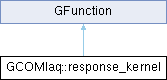GammaLib  1.7.0.dev
GCOMIaq::response_kernel Class Reference
Inheritance diagram for GCOMIaq::response_kernel:## Public Member Functions

response_kernel (const GCOMD1Response &response_d1, const GCOMD2Response &response_d2, const double &etrue1, const double &etrue2, const double &phibar, const double &etmin, const double &etmax, const double &e1min, const double &e1max, const double &e2min, const double &e2max)

double eval (const double &energy1)
Computes product of D1 and D2 responses at energy E1 and phibar. More...Public Member Functions inherited from GFunction
GFunction (void)
Void constructor. More...

GFunction (const GFunction &function)
Copy constructor. More...

virtual ~GFunction (void)
Destructor. More...

GFunctionoperator= (const GFunction &function)
Assignment operator. More...

## Protected Attributes

const GCOMD1Responsem_response_d1
Reference to D1 module response. More...

const GCOMD2Responsem_response_d2
Reference to D2 module response. More...

double m_etrue1
True D1 energy (MeV) More...

double m_etrue2
True D2 energy (MeV) More...

double m_cos_phibar
cos(phibar) More...

double m_sin_phibar
sin(phibar) More...

double m_etmin
Minimum total energy (MeV) More...

double m_etmax
Maximum total energy (MeV) More...

double m_e1min
Minimum D1 energy (MeV) More...

double m_e1max
Maximum D1 energy (MeV) More...

double m_e2min
Minimum D2 energy (MeV) More...

double m_e2max
Maximum D2 energy (MeV) More...Protected Member Functions inherited from GFunction
void init_members (void)
Initialise class members. More...

void copy_members (const GFunction &function)
Copy class members. More...

void free_members (void)
Delete class members. More...

## Detailed Description

Definition at line 97 of file GCOMIaq.hpp.

## Constructor & Destructor Documentation

 GCOMIaq::response_kernel::response_kernel ( const GCOMD1Response & response_d1, const GCOMD2Response & response_d2, const double & etrue1, const double & etrue2, const double & phibar, const double & etmin, const double & etmax, const double & e1min, const double & e1max, const double & e2min, const double & e2max )
inline

Definition at line 99 of file GCOMIaq.hpp.

## Member Function Documentation

 double GCOMIaq::response_kernel::eval ( const double & energy1 )
virtual

Computes product of D1 and D2 responses at energy E1 and phibar.

Parameters
 [in] energy1 D1 energy deposit (MeV).

The method computes

$R(E_1,E_2|\hat{E_1},\hat{E_2}) = R_1(E_1|\hat{E_1}) \times R_2(E_2|\hat{E_2}) \times J$

where $$R_1(E_1|\hat{E_1})$$ is the D1 module response function, $$R_2(E_2|\hat{E_2})$$ is the D2 module response function, $$E_1$$ and $$E_2$$ are the measured D1 and D2 energy deposits, respectively, $$\hat{E_1}$$ and $$\hat{E_2}$$ are the true D1 and D2 energy deposits, respectively, and $$J$$ is the Jacobian.

The measured D2 energy deposit is computed using

$E_2 = \frac{1}{2} \times \left( \sqrt{\frac{4 m_e c^2 E_1}{1 - \cos \bar{\varphi}} + E_1^2} - E_1 \right)$

where $$m_e\,c^2$$ the electron rest mass, and $$\bar{\varphi}$$ the Compton scatter angle.

The Jacobian is given by

$J = \frac{m_e c^2 \sqrt{E_1} \sin \bar{\varphi}} {(1 - \cos \bar{\varphi})^2 \sqrt{\frac{4 m_e c^2}{1 - \cos \bar{\varphi}} + E_1}}$

The code implementation is based on the COMPASS RESPSIT2 function FUNC.F (release 4.0, 21-Oct-91).

Implements GFunction.

Definition at line 1366 of file GCOMIaq.cpp.

## Member Data Documentation

 double GCOMIaq::response_kernel::m_cos_phibar
protected

cos(phibar)

Definition at line 128 of file GCOMIaq.hpp.

Referenced by eval().

 double GCOMIaq::response_kernel::m_e1max
protected

Maximum D1 energy (MeV)

Definition at line 133 of file GCOMIaq.hpp.

Referenced by eval().

 double GCOMIaq::response_kernel::m_e1min
protected

Minimum D1 energy (MeV)

Definition at line 132 of file GCOMIaq.hpp.

Referenced by eval().

 double GCOMIaq::response_kernel::m_e2max
protected

Maximum D2 energy (MeV)

Definition at line 135 of file GCOMIaq.hpp.

Referenced by eval().

 double GCOMIaq::response_kernel::m_e2min
protected

Minimum D2 energy (MeV)

Definition at line 134 of file GCOMIaq.hpp.

Referenced by eval().

 double GCOMIaq::response_kernel::m_etmax
protected

Maximum total energy (MeV)

Definition at line 131 of file GCOMIaq.hpp.

Referenced by eval().

 double GCOMIaq::response_kernel::m_etmin
protected

Minimum total energy (MeV)

Definition at line 130 of file GCOMIaq.hpp.

Referenced by eval().

 double GCOMIaq::response_kernel::m_etrue1
protected

True D1 energy (MeV)

Definition at line 126 of file GCOMIaq.hpp.

Referenced by eval().

 double GCOMIaq::response_kernel::m_etrue2
protected

True D2 energy (MeV)

Definition at line 127 of file GCOMIaq.hpp.

Referenced by eval().

 const GCOMD1Response& GCOMIaq::response_kernel::m_response_d1
protected

Reference to D1 module response.

Definition at line 124 of file GCOMIaq.hpp.

Referenced by eval().

 const GCOMD2Response& GCOMIaq::response_kernel::m_response_d2
protected

Reference to D2 module response.

Definition at line 125 of file GCOMIaq.hpp.

Referenced by eval().

 double GCOMIaq::response_kernel::m_sin_phibar
protected

sin(phibar)

Definition at line 129 of file GCOMIaq.hpp.

Referenced by eval().

The documentation for this class was generated from the following files: